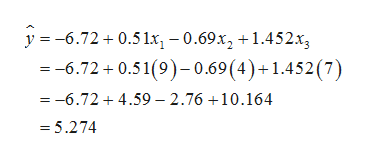Consider the following multiple regression model :         y hat = -6.72 + 0.51x1 - 0.69x2 + 1.452x3a. What is the mean value of y when x1 = 9, x2 = 4, and x3 = 7?b. write a sentence interpreting the value of ℬ2 hat = -0.69.c. Would the mean value of y be larger or smaller if x2 wereincreased, but x1 and x3 were held constant? Explain in a few sentences.d. If there were an interaction between x2 and x3, what additional predicator variable should be added to the model?

Question

Consider the following multiple regression model :

y hat = -6.72 + 0.51x1 - 0.69x2 + 1.452x3

a. What is the mean value of y when x1 = 9, x2 = 4, and x3 = 7?

b. write a sentence interpreting the value of ℬ2 hat = -0.69.

c. Would the mean value of y be larger or smaller if x2 wereincreased, but x1 and x3 were held constant? Explain in a few sentences.

d. If there were an interaction between x2 and x3, what additional predicator variable should be added to the model?

Step 1

The given multiple regression model is as follows:

Step 2

Calculations for Part (a):

It is given that the value of x1 is 9, the value of x2 is 4, and the value of x3 is 7.

The mean value of y is calculated as follows:help_outlineImage Transcriptionclosey 6.72 0.51x-0.69x2 +1.452x --6.72 0.51(9)-0.69 (4)+ 1.452 (7) =-6.72 4.59 2.76 +10.164 =5.274 fullscreen
Step 3

Interpretation of β2hat:

Significance of the coefficient:

In a multiple regression function, the coefficient corresponding to a particular explanatory variable signifies the effect of that explanatory variable on the response variable, while the other explanatory variables are kept at a fixed level.

The slope gives the amount of change in the response variable for unit increase in the explanatory variable. A positive slope implies that the response variable increases with increase in the explanatory variable, whereas a negat...

Want to see the full answer?

See Solution

Want to see this answer and more?

Our solutions are written by experts, many with advanced degrees, and available 24/7

See Solution
Tagged in

Statistics